3.MD.5.B.-Test1B A plane figure which can be covered by n unit squares is said
 Name:    3.MD.5.B.-Test1B A plane figure which can be covered by n unit squares

Multiple Choice
Identify the choice that best completes the statement or answers the question.

1.

How many square units does the shape have?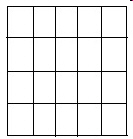a. 5 c. 20 b. 25 d. 4

2.

How many square units does the object have?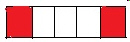a. 5 c. 3 b. 7 d. 2

3.

How many square units are in the object?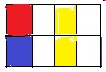a. 6 c. 4 b. 12 d. 8

4.

How many square units does the object have?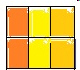a. 6 c. 12 b. 1 d. 24

5.

How many square units does the desktop have?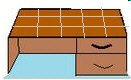a. 15 c. 3 b. 12 d. 5

6.

How many square units does the front of the box have?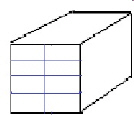a. 10 c. 18 b. 8 d. 12

7.

How many square units does Alice's closet have?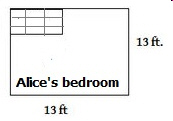a. 3 c. 13 b. 10 d. 9

8.

How many square units without the closet does Alice's bedroom have?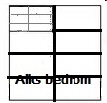a. 9 c. 20 b. 7 d. 6

9.

How many square units does the diagram have?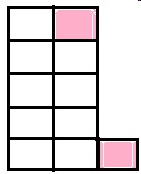a. 11 c. 20 b. 15 d. 9

10.

How many square units does the big square have?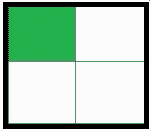a. 2 c. 4 b. 8 d. 12